Get instant live expert help with Excel or Google Sheets“My Excelchat expert helped me in less than 20 minutes, saving me what would have been 5 hours of work!”

#### Post your problem and you’ll get expert help in seconds.

Your message must be at least 40 characters
Our professional experts are available now. Your privacy is guaranteed.

# How to find moving average in Excel – Excelchat

Moving average in Excel (also known as rolling average, moving mean, or running average) is basically a series of calculated averages for varying subsets of the same set of data.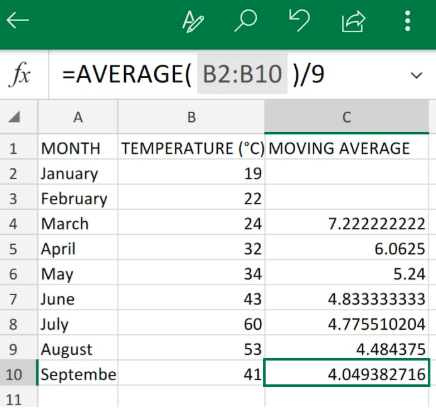Figure 1. Moving Average in Excel

## What is Moving Average in Excel?

The Excel Moving average formula is a popular time series analysis technique which is used for predicting the future. The moving/rolling average of a series of time values are essentially generated by calculating the averages of various times series of data.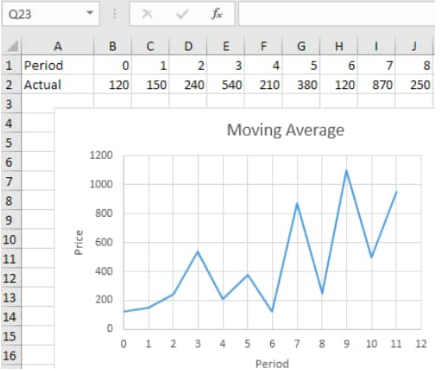Figure 2. Chart Representation of Moving Average in Excel

## How to Calculate Moving Average in Excel

We will demonstrate how we can calculate rolling average in Excel with the following simple steps;

Let’s assume that we are required to determine the moving average in Excel for temperatures over a 3 month period. We can calculate rolling average by determining the average value of temperatures between January and March, and then the average value of temperatures between February and April, and then, between March and May, etc.

1. We begin by collecting the data available to us inside our spreadsheet;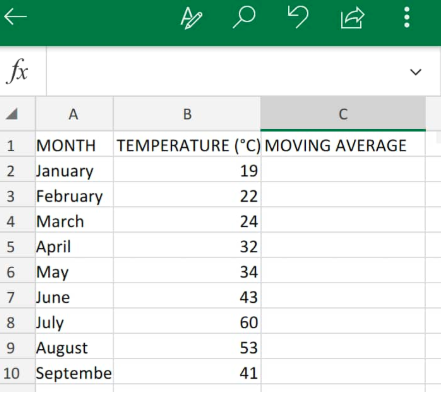Figure 3. Data Values in Excel

We can easily calculate the moving average by using the AVERAGE function in Excel.

1. We will input an Excel AVERAGE formula factoring in the initial 3 months in the row which corresponds to the third value (cell C4) from the top in our spreadsheet example.

The AVERAGE formula we will enter into cell C4 is as follows;

`=AVERAGE(B2:B4)/3`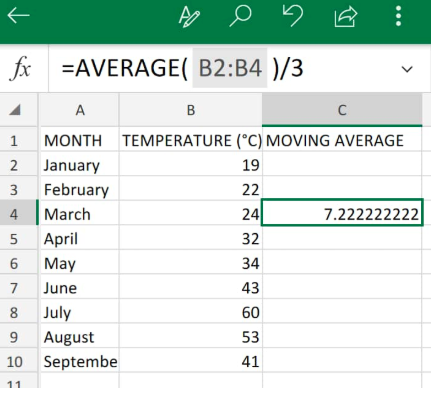Figure 4. Moving Average in Excel

Our Excel AVERAGE formula returned the rolling average value for temperatures in the first 3 months as 7.2.

1. Modify and copy the Excel Moving Average formula in C4 above down into the other cells in column C to get similar moving average in Excel results: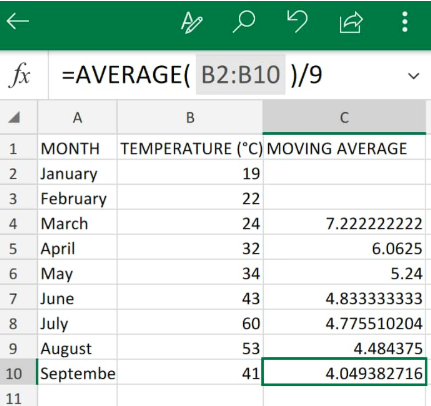Figure 5. Moving Average in Excel

## Instant Connection to an Excel Expert

Most of the time, the problem you will need to solve will be more complex than a simple application of a formula or function. If you want to save hours of research and frustration, try our live Excelchat service! Our Excel Experts are available 24/7 to answer any Excel question you may have. We guarantee a connection within 30 seconds and a customized solution within 20 minutes.